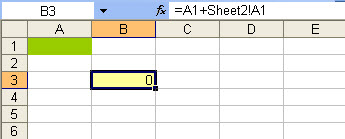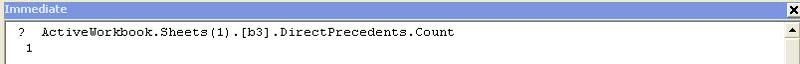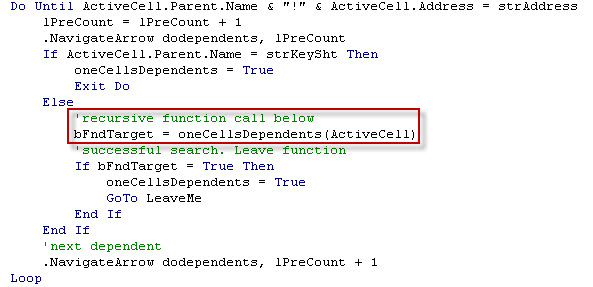# Using a recursive NavigateArrows Method to analyse all local and off sheet cell dependencies

Published on
10,374 Points
3,474 Views
4 Endorsements
Last Modified:
Approved
Community Pick

## Introduction

I do apologise for the academic sounding title, I simply couldn't find a better descriptor without the jargon. :)

This article revisits one of my favourite questions, http://www.experts-exchang­e.com/Q_23­787032.htm­l.

In short:
a list of numeric codes are contained in column A of the first worksheet, "Trial Balance"

other worksheets may use these codes within worksheet formulae (within formulae is an important distinction)

the Asker wanted to know which of these codes are not eventually referenced in the final worksheet, ""BS Movement"

## Outlining an approach

This was an interesting problem from a coding viewpoint, the actual key coding blocks were easily definable, but the solution would require:
1) searching and parsing strings,
2) working with off sheet references (3D functionality in Excel is not always robust and cell precedents/dependents are no exception),
3) recursion

Attached is the sample file, Sample-file.xls

## Main Subroutine

The first routine is a simple block of code that sets up the working range on the first sheet,  loops through and tests all non-empty calls for the referencing using the CellUsedinFormula routine, and then tidies up by removing the audit arrows and highlighting any non-referenced cells with a red background.

## CellUsedinFormula Subroutine

This routine searches each worksheet other than "Trial Balance" for a partial string match for the numeric code items.

## ExactMatch Function

When a match is found a regular expression user defined function (UDF), ExactMatch, is used to extract all numeric strings from the formula of interest. These strings are then subjected to a binary StrCompare to ensure that the match is exact, ie to prevent the false matching of "1000" when searching for "100".

A successful StrCompare means that we have identified a formula cell containing the numeric code from the "Trial Balance" sheet. If this cell resides in "BS Movement" then this code can be immediately ticked off the list, if not then a complete recursive check is required for all cells in the ActiveWorkbook that have a dependency on this cell formula. If any of this cells are located on the "BS Movement" sheet then we have a valid numeric code.

## oneCellsDependents Function

The recursive dependency check is run using the oneCellsDependents UDF.

Cell precedents and dependents is one of those areas where Excel VBA is decidedly worksheet centric. The basic sample below shows that B3 in Sheet1 is dependant on both A1 of Sheet1 (same sheet) and A1 from Sheet2 (off sheet).A simple test in the Immediate window of VBE shows that Excel sees only 1 DirectPrecedent (the same answer applies test for Precedent).So there is not a readymade collection of precedent (or in our particular example dependent) cells for us to apply a recursive search to.

Fortunately as per normal there is a workaround. Excel VBA provides us with the NavigateArrow Method, the VBA equivalent of the auditing toolbar found in 2003. This approach can be used to step though each of a cells dependents (or precedents) in turn by simulating clicking on the dependant arrow to each internal or off-sheet cell dependent.

Please note that the original credit for the navigate code that I modified below belongs to Bill Manville, Bill's original version  reference here was written to list all precedents of the active cell.

We now have the code blocks in place to identify cells of interest, and to test all the immediate dependencies of this cell to see if these cells are located on the "BS Movement"  worksheet. The remaining step is to broaden the dependency tracing code to handle a dependency chain of two or more cells. This is performed using a recursive programming technique, in plain English the oneCellsDependents calls itself until it either finds a dependent cell on the "BS Movement"  worksheet, or until the search has exhausted all the dependent cells without finding a match.## Other

Please note that I have used case-insensitive searching and comparison throughout this example so that the code could be easily modified for a text based search. See the code for the notes as to where to change this.

For more information on using Regular Expressions in VBA please see Patrick Matthew's excellent article,   Using Regular Expressions in Visual Basic.

I have made some minor modifications to the code from http://www.experts-exchang­e.com/Q_23­787032.htm­l  that is attached below.

``````Public Const strSourceSht = "Trial Balance"
Public Const strKeySht = "BS Movement"

Sub Main()
Dim ws As Worksheet
Dim rng1 As Range
Dim rng2 As Range
Dim i As Long
Set ws = ThisWorkbook.Sheets(strSourceSht)
Set rng1 = ws.Range(ws.[a2], ws.Cells(Rows.Count, "A").End(xlUp))
If rng1 Is Nothing Then Exit Sub
rng1.EntireColumn.Interior.ColorIndex = xlNone
Application.ScreenUpdating = False
For Each rng2 In rng1
If Len(rng2.Value) > 0 Then
rng2.NumberFormat = "@"
If CellUsedinFormula(Trim(rng2.Value)) Then
Else
i = i + 1
rng2.Interior.Color = vbRed
End If
End If
Next
For Each ws In ActiveWorkbook.Worksheets
ws.ClearArrows
Next ws
Application.Goto rng1.Cells(1)
Application.ScreenUpdating = True
If i > 0 Then MsgBox i & " cells were found that are not used" & vbNewLine & "these have been coloured red"
End Sub

Function CellUsedinFormula(strFormula As String) As Boolean
Dim ws As Worksheet
Dim rng1 As Range
Dim rng2 As Range
Dim strTest As String
Dim strFirst As String
Dim bFound As Boolean

'Look in each worksheet for the strings in the list from "Trial Balance"
For Each ws In ThisWorkbook.Worksheets
If ws.Name <> strSourceSht Then
On Error Resume Next
Set rng1 = ws.Cells.SpecialCells(xlCellTypeFormulas)
On Error GoTo 0
'Look in formula cells only
If Not rng1 Is Nothing Then
'the string searches and later string comparisons are case insenstive
'so that the code can be easily modified to look for text rather than numeric strings
Set rng2 = rng1.Find(strFormula, , xlFormulas, xlPart, xlByRows, , False)
'For a case senstive search make the last argument TRUE, ie
'Set rng2 = rng1.Find(strFormula, , xlFormulas, xlPart, xlByRows, , TRUE)

'If the string is found, used a regular expression UDF to test for an exact match
If Not rng2 Is Nothing Then
strTest = LCase\$(strFormula)
bFound = ExactMatch(strTest, rng2)
If bFound Then Exit For
strFirst = rng2.Address
Do
Set rng2 = rng1.FindNext(rng2)
bFound = ExactMatch(strTest, rng2)
'If a valid reference is found then stop the search for the string
If bFound Then Exit For
Loop While Not rng2 Is Nothing And rng2.Address <> strFirst
Exit For
End If
End If
End If
Next
CellUsedinFormula = bFound
End Function
Function ExactMatch(ByVal strTest, ByVal rng2) As Boolean
Dim regex As Object
Dim regM As Object
Dim RegC As Object
Dim bDependentRange As Boolean
Set regex = CreateObject("vbscript.regexp")
regex.Pattern = "\d+"
regex.Global = True
Set regM = regex.Execute(rng2.Formula)
For Each RegC In regM
If StrComp(LCase\$(strTest), RegC, vbBinaryCompare) = 0 Then
If rng2.Parent.Name = strKeySht Then
ExactMatch = True
Else
'Call the recursive dependents function to test if this string
'is eventually referred to on P&L Forecast
bDependentRange = oneCellsDependents(rng2)
If bDependentRange Then
'exit on a successful match
ExactMatch = True
Exit Function
End If
End If
End If
Next
End Function

Function oneCellsDependents(ByVal rng2) As Boolean
'initial code credited to Bill Manville
'brettdj adapted this code from a post by mike rickson
Dim strAddress As String
Dim rngReturn As Range
Dim i As Long
Dim lPreCount As Long
Dim bFndTarget As Boolean

'set the point to return to
Set rngReturn = Selection
strAddress = rng2.Parent.Name & "!" & rng2.Address

' use the NavigateArrow method to follow cell dependencies
With rng2
.ShowDependents
.NavigateArrow dodependents, 1
'recursive loop through dependents
Do Until ActiveCell.Parent.Name & "!" & ActiveCell.Address = strAddress
lPreCount = lPreCount + 1
.NavigateArrow dodependents, lPreCount
If ActiveCell.Parent.Name = strKeySht Then
oneCellsDependents = True
Exit Do
Else
'recursive function call below
bFndTarget = oneCellsDependents(ActiveCell)
'successful search. Leave function
If bFndTarget = True Then
oneCellsDependents = True
GoTo LeaveMe
End If
End If
'next dependent
.NavigateArrow dodependents, lPreCount + 1
Loop
If oneCellsDependents Then GoTo LeaveMe
'remove the auditing arrows
ActiveCell.ShowDependents Remove:=True
End With
'Return selection to where it was
With rngReturn
.Parent.Activate
.Select
End With
LeaveMe:
End Function
``````

=-=-=-=-=-=-=-=-=-=-=-=-=-=-=-=-=-=-=-=-=-=-=-=-=-=-=-=-=-=-=-=-=-=-=-=-=-=-=-=-=
If you liked this article and want to see more from this author, please click here.

If you found this article helpful, please click the Yes button near the:

Was this article helpful?

label that is just below and to the right of this text.   Thanks!
=-=-=-=-=-=-=-=-=-=-=-=-=-=-=-=-=-=-=-=-=-=-=-=-=-=-=-=-=-=-=-=-=-=-=-=-=-=-=-=-=
4
Ask questions about what you read
If you have a question about something within an article, you can receive help directly from the article author. Experts Exchange article authors are available to answer questions and further the discussion.
Get 7 days free